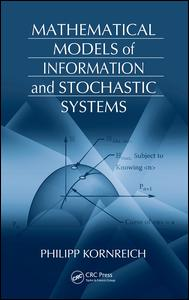# Mathematical Models of Information and Stochastic Systems

Regular price \$130.00 \$130.00 Sale

Author(s): Philipp Kornreich

From ancient soothsayers and astrologists to today’s pollsters and economists, probability theory has long been used to predict the future on the basis of past and present knowledge. Mathematical Models of Information and Stochastic Systems shows that the amount of knowledge about a system plays an important role in the mathematical models used to foretell the future of the system. It explains how this known quantity of information is used to derive a system’s probabilistic properties.

After an introduction, the book presents several basic principles that are employed in the remainder of the text to develop useful examples of probability theory. It examines both discrete and continuous distribution functions and random variables, followed by a chapter on the average values, correlations, and covariances of functions of variables as well as the probabilistic mathematical model of quantum mechanics. The author then explores the concepts of randomness and entropy and derives various discrete probabilities and continuous probability density functions from what is known about a particular stochastic system. The final chapters discuss information of discrete and continuous systems, time-dependent stochastic processes, data analysis, and chaotic systems and fractals.

By building a range of probability distributions based on prior knowledge of the problem, this classroom-tested text illustrates how to predict the behavior of diverse systems. A solutions manual is available for qualifying instructors.

Review(s):

EAN: 9781420058840